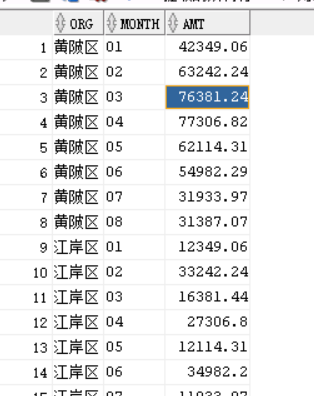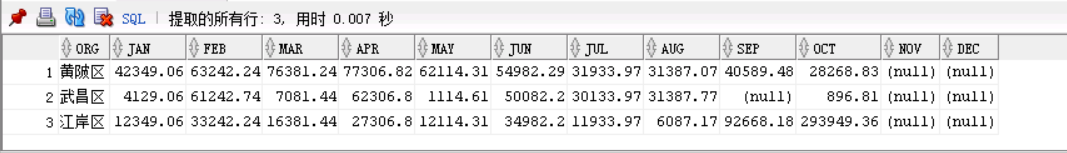WHCSRL 技术网

# Oracle pivot 行转列

### 1：数据准备

``create table t_sale (    org varchar(10),    month varchar(10),    amt number) Insert into t_sale (ORG,MONTH,AMT) values ('黄陂区','01',42349.06);Insert into t_sale (ORG,MONTH,AMT) values ('黄陂区','02',63242.24);Insert into t_sale (ORG,MONTH,AMT) values ('黄陂区','03',76381.24);Insert into t_sale (ORG,MONTH,AMT) values ('黄陂区','04',77306.82);Insert into t_sale (ORG,MONTH,AMT) values ('黄陂区','05',62114.31);Insert into t_sale (ORG,MONTH,AMT) values ('黄陂区','06',54982.29);Insert into t_sale (ORG,MONTH,AMT) values ('黄陂区','07',31933.97);Insert into t_sale (ORG,MONTH,AMT) values ('黄陂区','08',31387.07);Insert into t_sale (ORG,MONTH,AMT) values ('江岸区','01',12349.06);Insert into t_sale (ORG,MONTH,AMT) values ('江岸区','02',33242.24);Insert into t_sale (ORG,MONTH,AMT) values ('江岸区','03',16381.44);Insert into t_sale (ORG,MONTH,AMT) values ('江岸区','04',27306.8);Insert into t_sale (ORG,MONTH,AMT) values ('江岸区','05',12114.31);Insert into t_sale (ORG,MONTH,AMT) values ('江岸区','06',34982.2);Insert into t_sale (ORG,MONTH,AMT) values ('江岸区','07',11933.97);Insert into t_sale (ORG,MONTH,AMT) values ('江岸区','08',6087.17);Insert into t_sale (ORG,MONTH,AMT) values ('武昌区','01',4129.06);Insert into t_sale (ORG,MONTH,AMT) values ('武昌区','02',61242.74);Insert into t_sale (ORG,MONTH,AMT) values ('武昌区','03',7081.44);Insert into t_sale (ORG,MONTH,AMT) values ('武昌区','04',62306.8);Insert into t_sale (ORG,MONTH,AMT) values ('武昌区','05',1114.61);Insert into t_sale (ORG,MONTH,AMT) values ('武昌区','06',50082.2);Insert into t_sale (ORG,MONTH,AMT) values ('武昌区','07',30133.97);Insert into t_sale (ORG,MONTH,AMT) values ('武昌区','08',31387.77);Insert into t_sale (ORG,MONTH,AMT) values ('黄陂区','09',40589.48);Insert into t_sale (ORG,MONTH,AMT) values ('江岸区','09',92668.18);Insert into t_sale (ORG,MONTH,AMT) values ('江岸区','10',293949.36);Insert into t_sale (ORG,MONTH,AMT) values ('黄陂区','10',28268.83);Insert into t_sale (ORG,MONTH,AMT) values ('武昌区','10',896.81);``### 2: pivot函数语法

``SELECT <non-pivoted column>,      [first pivoted column] AS <column name>,      [second pivoted column] AS <column name>,      ...      [last pivoted column] AS <column name>  FROM      (<SELECT query that produces the data>)       AS <alias for the source query>  PIVOT  (      <aggregation function>(<column being aggregated>)  FOR   [<column that contains the values that will become column headers>]       IN ( [first pivoted column], [second pivoted column],      ... [last pivoted column])  ) AS <alias for the pivot table>  <optional ORDER BY clause>;``

其中：

<aggregation function>(<column being aggregated>)

FOR

[<column that contains the values that will become column headers>]  行转列的标准

IN ( [first pivoted column], [second pivoted column],   行转列取值
... [last pivoted column])

``PIVOT ( sum(AMT)  FOR month  IN ('01' as Jan,'02' as Feb,'03' as Mar,                '04' as Apr,'05' as May,'06' as Jun,                '07' as Jul,'08' as Aug,'09' as Sep,                '10' as Oct,'11' as Nov,'12' as Dec))``

### 3：SQL实现如下：

``select * from (select org,month,amt  from  t_sale)pivot ( sum(amt)  for month  in ('01' as jan,'02' as feb,'03' as mar,                     '04' as apr,'05' as may,'06' as jun,                     '07' as jul,'08' as aug,'09' as sep,                     '10' as oct,'11' as nov,'12' as dec))``